By Roland Wenzlofsky

May 4, 2017

Heuristics are applied whenever there are no collected statistics available for a nonindexed column. Heuristics are rules of thumb to estimate the number of selected data rows. They are used in WHERE condition predicates and to join planning.

Heuristics estimate the number of selected data rows to be a certain percentage of the table cardinality. Maybe you wonder, how the optimizer knows the table cardinality?

The optimizer can use collected summary statistics or collected primary index statistics to estimate table cardinality. In the absence of collected statistics, the optimizer still can retrieve a random AMP sample to estimate table cardinality.

All heuristic estimations done by the Teradata Optimizer are based on the estimated table cardinality.
In this post, we will analyze several queries, and their estimates based on heuristics. Furthermore, we will learn how to replace heuristic estimations with more accurate ones; we want to avoid heuristic estimates, as they are only providing inaccurate statistical information to the Teradata Optimizer.

Statistics on nonindexed columns play a significant role in WHERE condition predicates. We will start with very simple examples to teach the base concepts of heuristics and will introduce more complex setups afterward.

Before we dive deeper into the details of heuristics, one important note:

Don’t forget: The following information is related to nonindexed columns. Whenever we refer to the “number of rows selected” or a “percentage of rows selected,” the table row estimation is based on collected summary or index statistics, or on a random AMP sample. Just keep in mind, when we refer to table row counts, that we mean the estimations, not real numbers.

Just keep in mind, when we refer to table row counts, that we mean the estimations, not real table row numbers.
Here is the test table we will use in all our examples. The table row count is 100.000, which is the base for all heuristic estimations the optimizer is doing.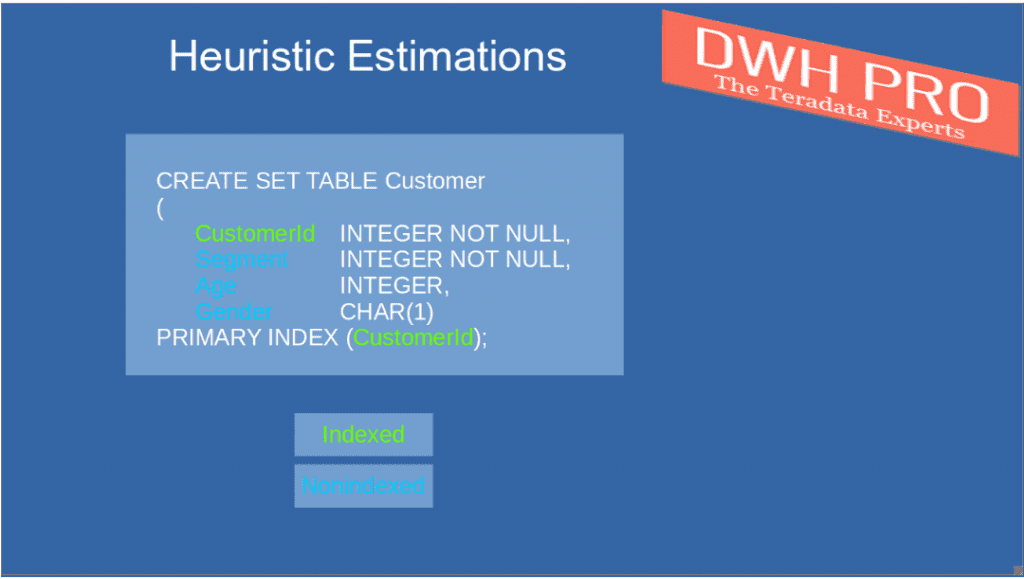￼￼
We will start our tour with a very simple example, having only one WHERE condition predicate for a nonindexed column (”segment” is neither primary index nor any other index of the customer table)

Here is our test query: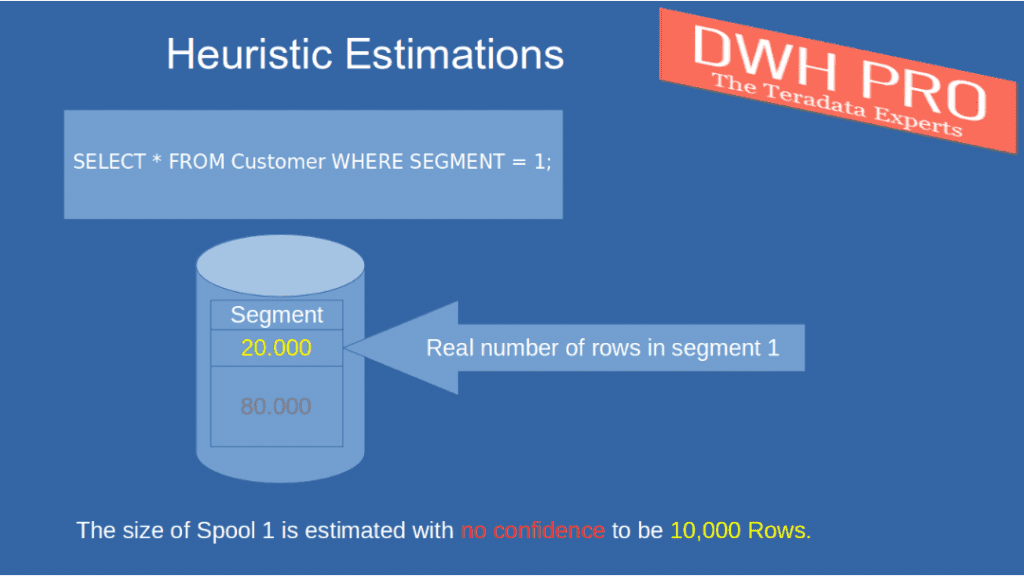We collected statistics on the primary index column of table “customer”. The row count estimation derived from these statistics is 100.000.

We did not collect statistics on the nonindexed column “segment”

The real number of rows in “segment 1” is 20.000.

As the column “segment” is a nonindexed column, the optimizer applies heuristic estimations.

The number of selected rows is estimated to be 10% of the table rows.

The hard coded rule is 10% without any exception

100.000 * 10% = 10.000 rows

The optimizer underestimated the real number of data rows by 50%! If we would have 0 rows in segment 1, the estimation would still be 10.000 rows, and in this case, the optimizer would drastically overestimate the number of selected rows.

We consider above “10% Rule” as the base estimation the optimizer uses whenever there is only one WHERE condition predicate available for a single value.
Our first example carved out, why heuristic estimates should be avoided at all cost: They are often far away from reality.

In the next example, we will add another WHERE condition predicate for a second nonindexed column to the query.

We did not collect statistics on any of the columns used in the predicates. This is our test query:

SELECT * FROM Customer WHERE SEGMENT = 1 AND Age = 25;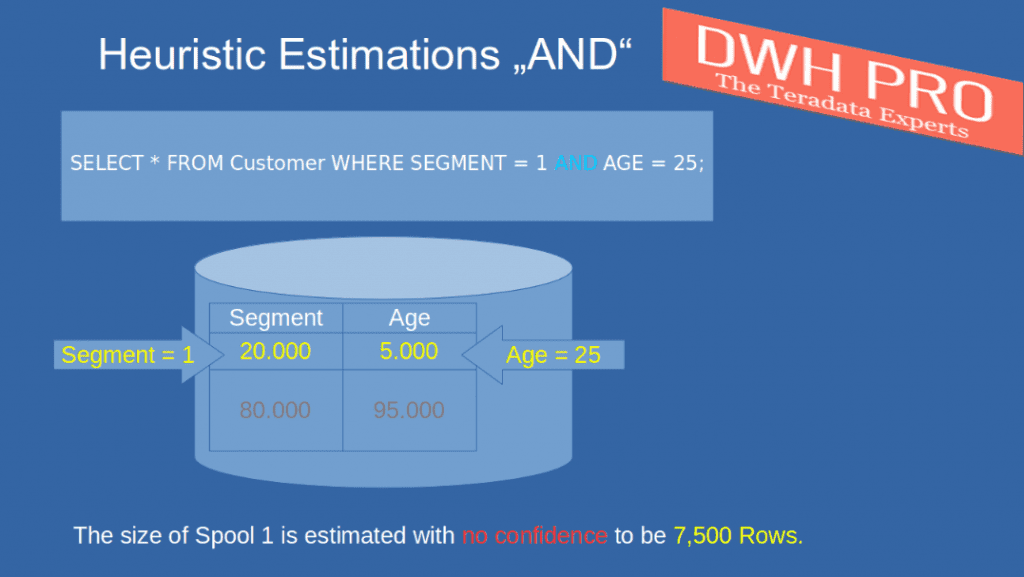The heuristic rule applied for nonindexed columns which are AND combined is:

For each AND combined predicate, we multiply the initial estimate by 75%.

Let’s calculate the estimation for our example query:

10% from 100.000 data rows = 10.000 rows. This is the base estimation. We multiply the base estimation by 75%, i.e. 10.000 * 75%. The result is 7.500 rows.

We could extend our example and add one more WHERE condition predicate, this would lead us to the following total estimate:

((100.000 * 10%) *75%) * 75% = 5.625 data rows.

Finally, by adding one more additional WHERE condition predicate, we would be down to 4.219 rows.

Each additional “AND”-combined WHERE condition predicate decreases the number of estimated rows: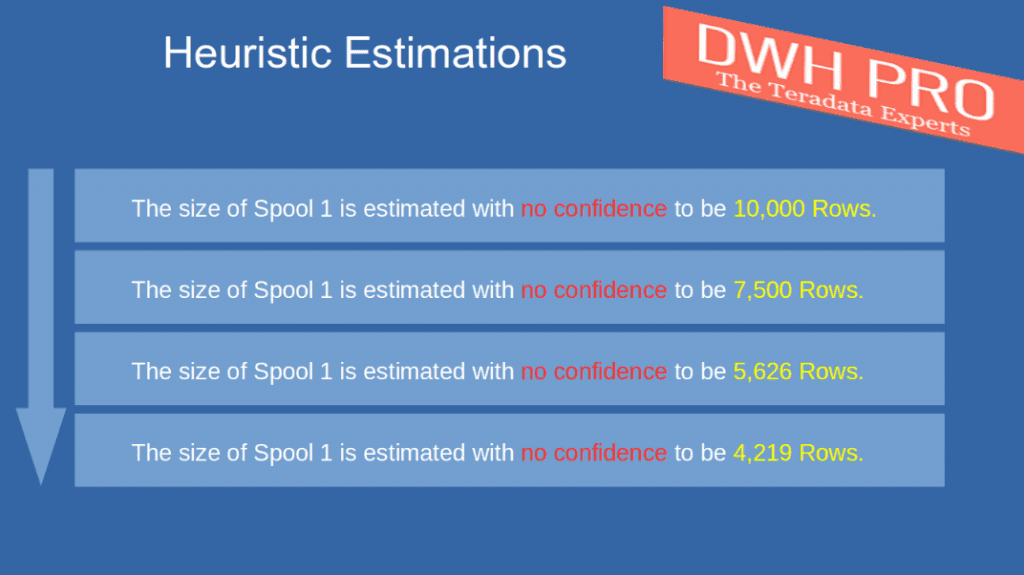In the next example, we will learn how heuristic estimates are implemented for WHERE condition predicates on different OR combined columns.
SELECT * FROM Customer WHERE SEGMENT = 1 OR Age = 25;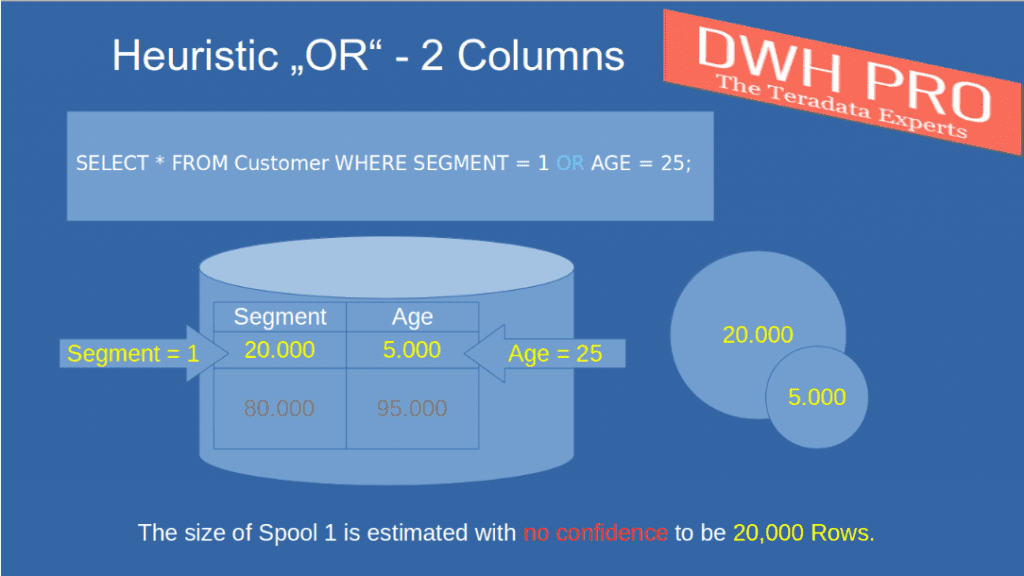The rule for OR combined predicates for different columns is as follows:

The estimate for each predicate is the base estimation we defined before (i.e. 10% of the table rows)

We know that the estimated number of rows in the customer table is 100.000.

The estimation for the result set of the query is (100.000 * 10%) + (100.000 * 10%), or 20.000 rows (2 times the base estimation)

Like in the previous example, estimations will be either too high or too low, and most probably never will match the real number of rows being selected.

Of course, there is an upper limit for the estimation. If we combine 11 conditions with “OR”, the estimate will not be 110%, but 100%.

These were the most simple heuristic rules, knowing them gives you already a very good insight, and they will help you to understand execution plan estimations.
The heuristic estimations for OR combined predicates on the same columns are more complex.

Nevertheless, we decoded the applied algorithm and carved out the basic rules and building blocks.

First of all, the optimizer distinguishes between ranges of values and single values.

SELECT * FROM Customer WHERE Age in (20,22,24);
SELECT * FROM Customer WHERE Age = 20 OR AGE = 22 OR Age = 24;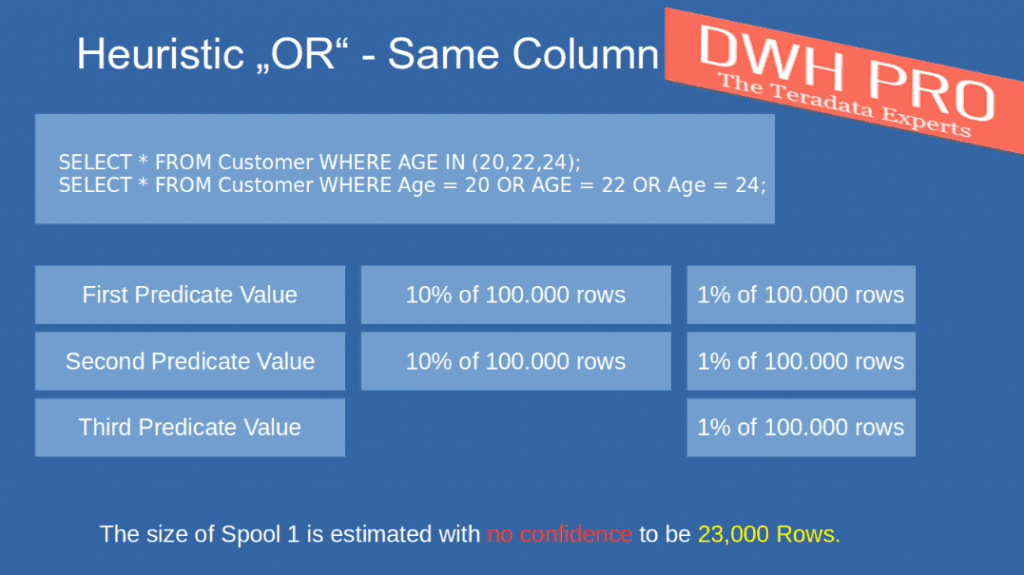The optimizer will assume that 10% of the table rows will be selected, but only for the first two predicates!

Starting with the second predicate, 1% of the table cardinality will be added for all predicates (including the first one). Starting with the third predicate, no base estimation (10% of table cardinality) will be added!
For our example this means:

(100.000 * 10%) for the first predicate
(100.000 * 10%) for the second predicate

(3 * (100.000 * 1%)) = 3.000 for the three predicates.

The total estimation, therefore, is 23.000 rows.

Estimations for Ranges of Values

Here are examples of range predicates. There is no gap between the selected values.

SELECT * FROM Customer WHERE Age in (20,21,22);
SELECT * FROM Customer WHERE Age BETWEEN 20 AND 22;
SELECT * FROM Customer WHERE Age = 20 OR AGE = 21 OR Age = 22;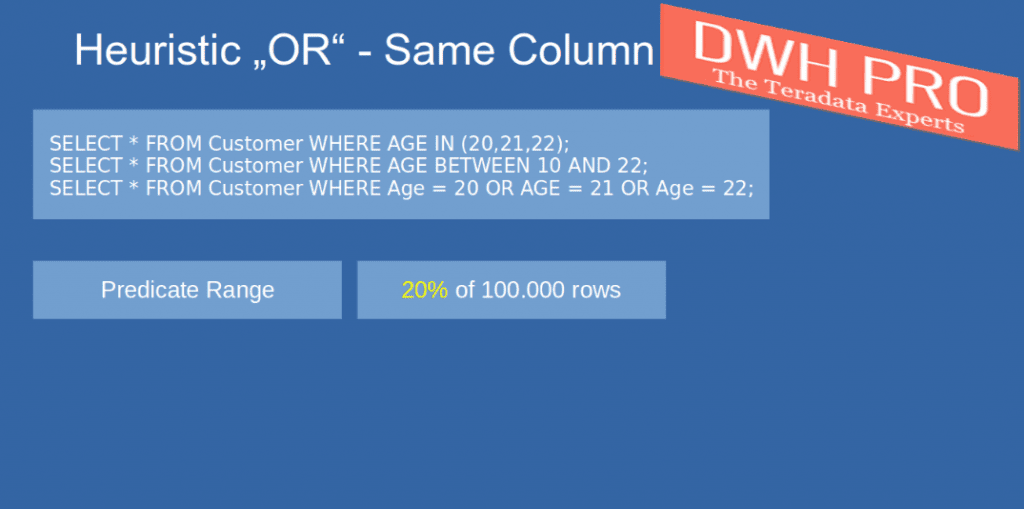If our WHERE condition contains only one range predicate, the estimation will be 20% of the table rows, in our example, this is 20.000 rows.

It doesn’t matter how many values the ranges contain. The estimation equally will be 20% for this query:

SELECT * FROM Customer WHERE Age BETWEEN 1 AND 1000;If we add a second range to our query, estimation rises to 2 times 20% or 40%: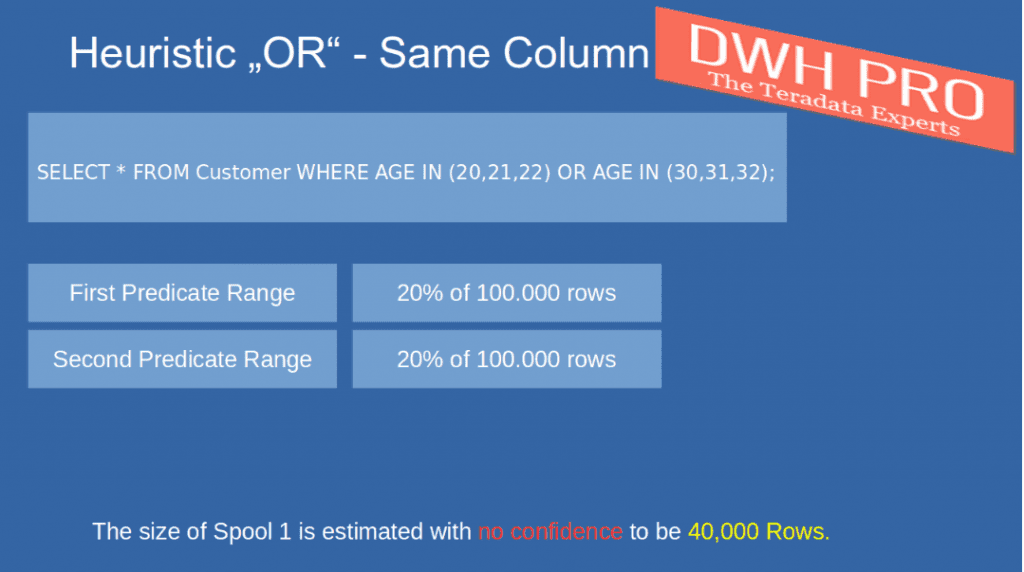If we add a third range, a more detailed estimation is applied.
The estimate for the first and second range predicate is each 10% (remember, in the previous example with to ranges, it was 20% per range predicate).

There will be no 10% estimations added after the second range predicate, but similarly to the estimations for single values, the number of distinct values in the range is counted and for each value, the estimation is increased by 1%.

SELECT * FROM Customer WHERE Age IN (10,11,12) OR AGE IN (20,21,22) OR AGE IN (30,31,32);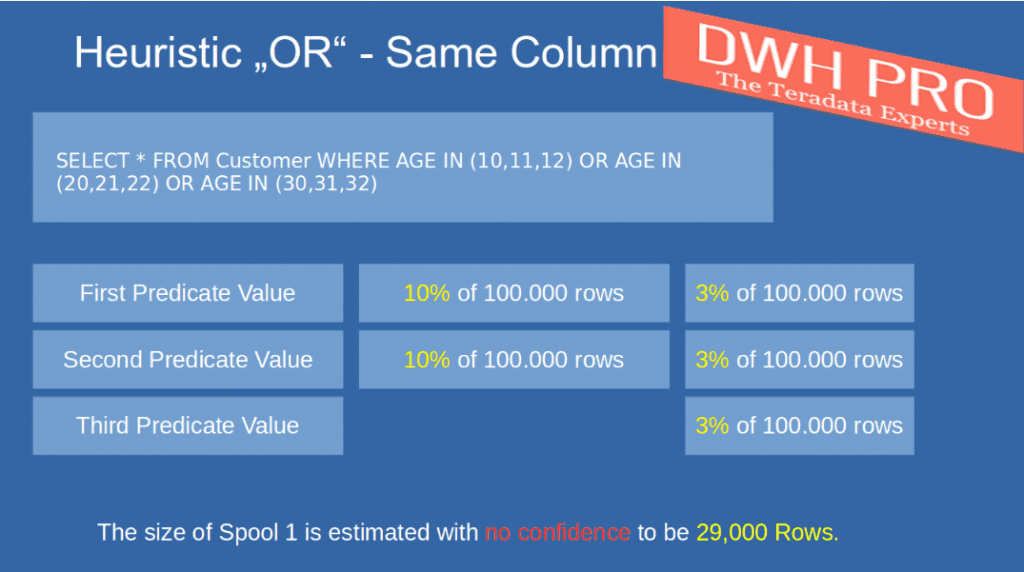For our example this means:

(100.000 * 10%) for the first range predicate
(100.000 * 10%) for the second range predicate

(9 * (100.000 * 1%)) = 9.000 for the nine values.

The total estimation, therefore, is 29.000 rows.

The estimation for one range predicate is always 20%, the number of distinct range values is not changing the estimation.

The estimation for two range predicates increases to 40%, and the number of distinct range values is not changing the estimation.

If our query has 3 or more range predicates, the estimation for the first 2 ranges is 20% (10% plus 10%), each distinct value, of all ranges, increases the estimation by 1%.
We will now come to the question: “What is the impact on heuristic estimations if we collect statistics one or more of the WHERE condition predicates”?

In this case, the optimizer considers all WHERE conditions with collected statistics and chooses the estimation of the one with the highest selectivity as the starting point for further estimations.

In other words: The estimations will not start with the usual heuristic estimation (10%, 20%, etc.), but with a much better estimation, based on collected statistics. Here is an example: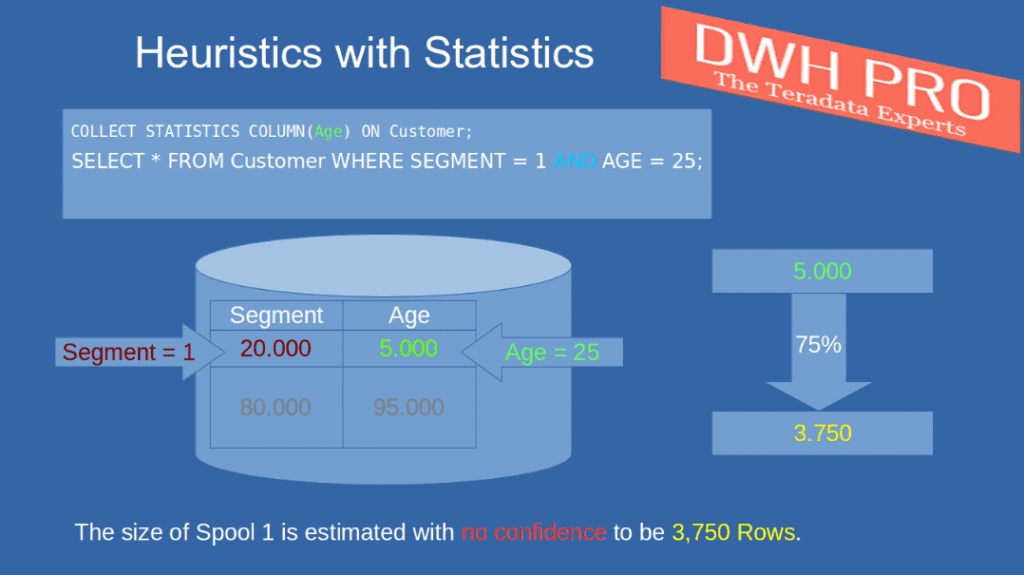IN above example, at first, we select statistics on column CustomerAge:

COLLECT STATISTICS COLUMN(Age) ON Customer;

We assume that the estimation for customers age 25 is 5.000 data rows.

We execute the select statement:

SELECT * FROM Customer WHERE Segment = 1 AND Age = 25;

Both columns are nonindexed.

As we collected on exactly one column statistics, they will be the starting point for the estimations, namely 5.000 data rows (the selectivity for CustomerAge=25)

Like in our previous example related to AND combined conditions, for the second column the optimizer will apply heuristics:

5.000 * 75% = 3.750 data rows.

Whenever there is at least one column with collected statistics available, this can drastically increase the quality of the estimation.
I have another example for you, this time we combine three columns with “AND”, two of them having collected statistics, one without statistics.

Of course, all columns, like always, are nonindexed columns. But I assume this is clear by now.

We collect statistic on two columns:
COLLECT STATISTICS COLUMN(CustomerAge) ON Customer;
COLLECT STATISTICS COLUMN(Gender) ON Customer;

The collected estimation for customer age is 5.000 rows, the estimation for Gender=’U’ (for value “unknown”) is just 100 rows.

Like in the previous example, the optimizer picks the column with the highest selectivity to make its initial estimation: In our example, his is 100 rows.

Although we have a second column with collected statistics, they are of no use to the optimizer: The second and thirst column estimations are again calculated with above 75% rule:

(100 * 75%) * 75% = 56.25 rows (which will be rounded to 57 rows).
SELECT * FROM Customer WHERE CustomerId = 1 AND CustomerAge = 25 AND Gender=’U’;

Here is the last example demonstrating a combination of “OR” combined columns with and without statistics (sorry, no graphic available):

We collect statistics on CustomerAge.

COLLECT STATISTICS COLUMN(CustomerAge) ON Customer;

SELECT * FROM Customer WHERE CustomerId = 1 OR CustomerAge = 25;

The optimizer will simply sum up estimates taken from collected statistics and heuristic estimates for columns without collected statistics:

Again, the estimation for CustomerAge=25 is 5.000 rows. Heuristics for CustomerId=1 give 10.000 rows (100.000 * 10%).

The total estimation is 15.000 rows.

We could go on forever with examples for heuristic estimations, but we will stop here with some final examples for frequently used WHERE condition predicates: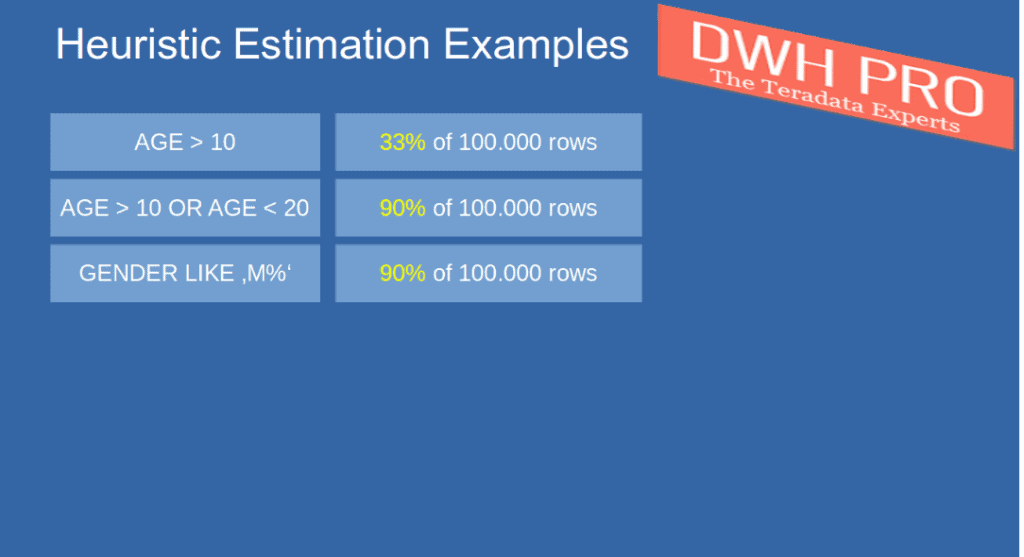•DWH Pro Admin says:

These are without collected statistics on AGE and GENDER

• Great article and examples.
I’ve got a question regarding the last frequently used WHERE conditions predicate example. Are these heuristic estimates based on a table which has no collected statistics on AGE and GENDER columns?